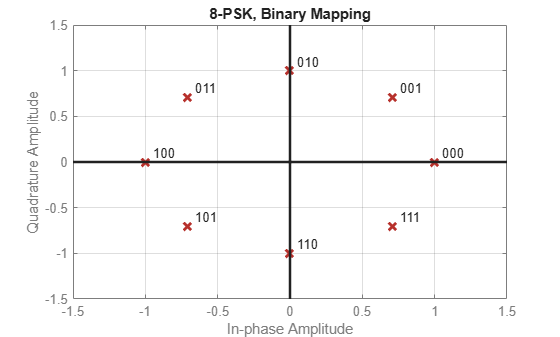# pskmod

Modulate signal using M-PSK method

## Syntax

``y = pskmod(x,M)``
``y = pskmod(x,M,phaseoffset)``
``y = pskmod(x,M,phaseoffset,symorder)``
``y = pskmod(x,M,Name=Value)``

## Description

````y = pskmod(x,M)` modulates the input signal `x` using the M-Ary phase shift keying (M-PSK) method. `M` specifies the modulation order.```

example

````y = pskmod(x,M,phaseoffset)` specifies the phase offset of the M-PSK constellation.```
````y = pskmod(x,M,phaseoffset,symorder)` specifies the symbol order of the M-PSK constellation.```
````y = pskmod(x,M,Name=Value)` specifies options using name-value arguments.```

## Examples

collapse all

Modulate and plot the constellations of QPSK and 16-PSK signals.

QPSK

Set the modulation order to 4.

`M = 4;`

Generate random data symbols.

`data = randi([0 M-1],1000,1);`

Modulate the data symbols.

`txSig = pskmod(data,M,pi/M);`

Pass the signal through white noise and plot its constellation.

```rxSig = awgn(txSig,20); scatterplot(rxSig)```16-PSK

Change the modulation order from 4 to 16.

`M = 16;`

Generate random data symbols.

`data = randi([0 M-1],1000,1);`

Modulate the data symbols.

`txSig = pskmod(data,M,pi/M);`

Pass the signal through white noise and plot its constellation.

```rxSig = awgn(txSig,20); scatterplot(rxSig)```Generate random symbols.

`dataIn = randi([0 3],1000,1);`

QPSK modulate the data.

`txSig = pskmod(dataIn,4,pi/4);`

Pass the signal through an AWGN channel.

`rxSig = awgn(txSig,10);`

Demodulate the received signal and compute the number of symbol errors.

```dataOut = pskdemod(rxSig,4,pi/4); numErrs = symerr(dataIn,dataOut)```
```numErrs = 2 ```

Set the modulation order, then create a data sequence containing a complete set of constellation points.

```M = 8; data = (0:M-1); phaseoffset = 0;```

Visualize the plot constellations of 8-PSK symbol mapping for modulated and demodulated gray and natural binary encoded data.

```symgray = pskmod(data,M,phaseoffset,'gray',PlotConstellation=true, ... InputType='integer');``````mapgray = pskdemod(symgray,M,phaseoffset,'gray',OutputType='integer'); symbin = pskmod(data,M,phaseoffset,'bin'); mapbin = pskdemod(symbin,M,phaseoffset,'bin',PlotConstellation=true, ... OutputType='bit');```## Input Arguments

collapse all

Input signal, specified as a scalar, vector, or matrix of positive integers. The elements of `x` must have binary or integer values in the range [0, `M`–1], where `M` is the modulation order.

Note

To process an input signal as binary elements, set the `InputType` name-value argument to `'bit'`. For binary inputs, the number of rows must be an integer multiple of log2(`M`). The function maps groups of log2(`M`) bits onto a symbol, with the first bit representing the MSB and the last bit representing the LSB.

Data Types: `double` | `single` | `int8` | `int16` | `int32` | `uint8` | `uint16` | `uint32` | `logical`

Modulation order, specified as an integer value greater than 1.

Data Types: `double`

Phase offset of the PSK constellation in radians, specified as a scalar.

Data Types: `double`

Symbol order, specified as `'gray'`, `'bin'`, or a vector. This argument specifies how the function assigns binary vectors to corresponding integers.

• `'gray'` — Use a Gray-coded ordering.

• `'bin'` — Use a natural binary-coded ordering.

• vector –– Use custom symbol ordering. The vector is of length `M` containing unique values in the range [0, `M`– 1]. The first element correlates to the constellation point corresponding to angle `phaseoffset`, with subsequent elements running counter-clockwise.

### Name-Value Arguments

Specify optional pairs of arguments as `Name1=Value1,...,NameN=ValueN`, where `Name` is the argument name and `Value` is the corresponding value. Name-value arguments must appear after other arguments, but the order of the pairs does not matter.

Before R2021a, use commas to separate each name and value, and enclose `Name` in quotes.

Example: ```y = pskmod(x,M,phaseoffset,symorder,InputType='bit')```

Input type, specified as either `'integer'` or `'bit'`.

• `'integer'` –– Input signal consists of integers in the range [0, `M`–1].

• `'bit'` –– Input signal consists of binary values and the number of rows must be an integer multiple of log2(`M`).

Output data type, specified as either `'double'` or `'single'`.

Option to plot constellation, specified as logical `0` (`false`) or `1` (`true`). To plot the PSK constellation, set `'PlotConstellation'` to `true`.

## Output Arguments

collapse all

M-PSK modulated baseband signal, returned as a scalar, vector or matrix of complex values. The columns of `y` represent independent channels. For integer inputs, the output `y` has the same dimensions as the input signal `x`. For bit inputs, the number of rows in `y` is the number of rows in `x` divided by log2(`M`).

 Proakis, John G. Digital Communications. 4th ed. New York: McGraw Hill, 2001.